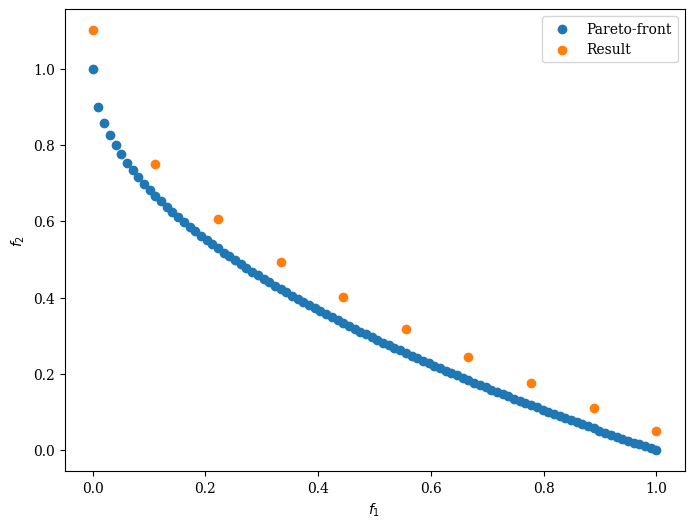Version: 0.5.0

Performance Indicator¶

It is fundamental for any algorithm to measure the performance. In a multi-objective scenario, we can not calculate the distance to the true global optimum but must consider a set of solutions. Moreover, sometimes the optimum is not even known, and other techniques must be used.

First, let us consider a scenario where the Pareto-front is known:

:
import numpy as np
from pymoo.factory import get_problem
from pymoo.visualization.scatter import Scatter

# The pareto front of a scaled zdt1 problem
pf = get_problem("zdt1").pareto_front()

# The result found by an algorithm
A = pf[::10] * 1.1

# plot the result
:
<pymoo.visualization.scatter.Scatter at 0x7f9261187c10>Generational Distance (GD)¶

The GD performance indicator  measure the distance from solution to the Pareto-front. Let us assume the points found by our algorithm are the objective vector set $$A=\{a_1, a_2, \ldots, a_{|A|}\}$$ and the reference points set (Pareto-front) is $$Z=\{z_1, z_2, \ldots, z_{|Z|}\}$$. Then,

\begin{align} \begin{split} \text{GD}(A) & = & \; \frac{1}{|A|} \; \bigg( \sum_{i=1}^{|A|} d_i^p \bigg)^{1/p}\\[2mm] \end{split} \end{align}

where $$d_i$$ represents the Euclidean distance (p=2) from $$a_i$$ to its nearest reference point in $$Z$$. Basically, this results in the average distance from any point $$A$$ to the closest point in the Pareto-front.

:
from pymoo.factory import get_performance_indicator

gd = get_performance_indicator("gd", pf)
print("GD", gd.do(A))
GD 0.05497689467314528

Generational Distance Plus (GD+)¶

Ishibushi et. al. proposed in  GD+:

\begin{align} \begin{split} \text{GD}^+(A) & = & \; \frac{1}{|A|} \; \bigg( \sum_{i=1}^{|A|} {d_i^{+}}^2 \bigg)^{1/2}\\[2mm] \end{split} \end{align}

where for minimization $$d_i^{+} = max \{ a_i - z_i, 0\}$$ represents the modified distance from $$a_i$$ to its nearest reference point in $$Z$$ with the corresponding value $$z_i$$.

:
from pymoo.factory import get_performance_indicator

gd_plus = get_performance_indicator("gd+", pf)
print("GD+", gd_plus.do(A))
GD+ 0.05497689467314528

Inverted Generational Distance (IGD)¶

The IGD performance indicator  inverts the generational distance and measures the distance from any point in $$Z$$ to the closest point in $$A$$.

\begin{align} \begin{split} \text{IGD}(A) & = & \; \frac{1}{|Z|} \; \bigg( \sum_{i=1}^{|Z|} \hat{d_i}^p \bigg)^{1/p}\\[2mm] \end{split} \end{align}

where $$\hat{d_i}$$ represents the euclidean distance (p=2) from $$z_i$$ to its nearest reference point in $$A$$.

:
from pymoo.factory import get_performance_indicator

igd = get_performance_indicator("igd", pf)
print("IGD", igd.do(A))
IGD 0.06690908300327662

Inverted Generational Distance Plus (IGD+)¶

In  Ishibushi et. al. proposed IGD+ which is weakly Pareto compliant wheres the original IGD is not.

\begin{align} \begin{split} \text{IGD}^{+}(A) & = & \; \frac{1}{|Z|} \; \bigg( \sum_{i=1}^{|Z|} {d_i^{+}}^2 \bigg)^{1/2}\\[2mm] \end{split} \end{align}

where for minimization $$d_i^{+} = max \{ a_i - z_i, 0\}$$ represents the modified distance from $$z_i$$ to the closest solution in $$A$$ with the corresponding value $$a_i$$.

:
from pymoo.factory import get_performance_indicator

igd_plus = get_performance_indicator("igd+", pf)
print("IGD+", igd_plus.do(A))
IGD+ 0.06466828842775944

Hypervolume¶

For all performance indicators showed so far, a target set needs to be known. For Hypervolume only a reference point needs to be provided. First, I would like to mention that we are using the Hypervolume implementation from DEAP. It calculates the area/volume, which is dominated by the provided set of solutions with respect to a reference point.This image is taken from  and illustrates a two objective example where the area which is dominated by a set of points is shown in grey. Whereas for the other metrics, the goal was to minimize the distance to the Pareto-front, here, we desire to maximize the performance metric.

:
from pymoo.factory import get_performance_indicator

hv = get_performance_indicator("hv", ref_point=np.array([1.2, 1.2]))
print("hv", hv.do(A))
hv 0.9631646448182305